## RS Aggarwal Class 8 Solutions Chapter 6 Operations on Algebraic Expressions Ex 6D

These Solutions are part of RS Aggarwal Solutions Class 8. Here we have given RS Aggarwal Solutions Class 8 Chapter 6 Operations on Algebraic Expressions Ex 6D.

Other Exercises

Question 1.
Solution:
(i)(x + 6)(x + 6)
= (x + 6)2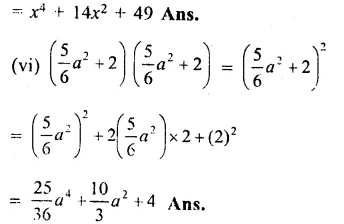Question 2.
Solution:
(i) (x – 4)(x – 4)
= (x – 4)2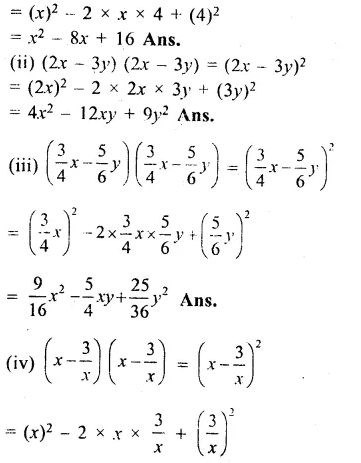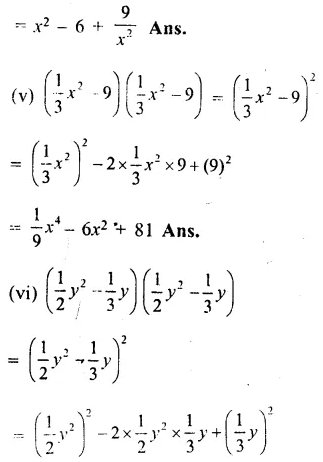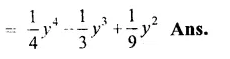Question 3.
Solution:
(i)(8a + 3b)2
= (8a)2 + 2 x 8a x 3b + (3b)2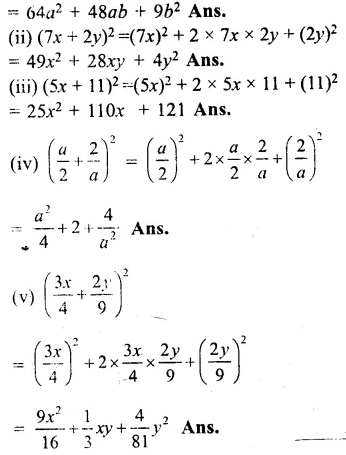Question 4.
Solution:
(i)(x + 3)(x – 3)
= (x)2 – (3)2Question 5.
Solution:
(i) (54)2
= (50 + 4)2Question 6.
Solution:
(i) (69)2
= (70 – 1)2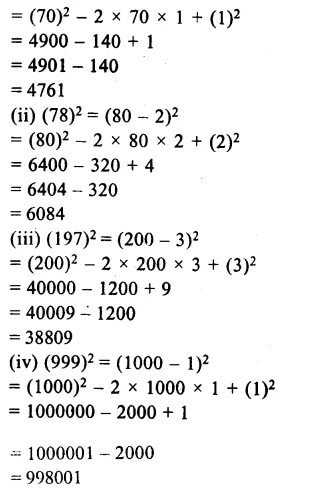Question 7.
Solution:
(i)(82)2 – (18)2
= (82 – 18)(82 – 18)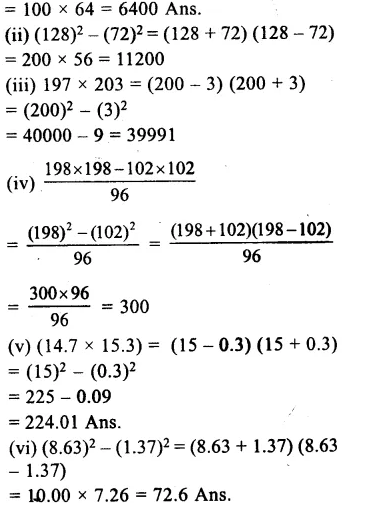Question 8.
Solution:
9x2 + 24x + 16
= (3x)2 + 2 x 3x x 4 + (4)2
= (3x + 4)2
= (3 x 12 + 4)2
= (36 + 4)2
= (40)2
= 1600 Ans.

Question 9.
Solution:
64x2 + 81y2 + 144xy = (8x)2 + (9y)2 + 2 x 8x x 9y
= (8x + 9y)2
= $${ \left( 8\times 11+9\times \frac { 4 }{ 3 } \right) }^{ 2 }$$
= (88 + 12)2
= (100)2
= 10000 Ans.

Question 10.
Solution:
(36x2 + 25y2 – 60xy)
= (6x)2 + (5y)2 – 2 x 6x x 5y
= (6x – 5y)2
= $${ \left( 6\times \frac { 2 }{ 3 } -5\times \frac { 1 }{ 5 } \right) }^{ 2 }$$
= (4 – 1)2
= (3)2 = 9

Question 11.
Solution:
$$\left( x+\frac { 1 }{ 4 } \right) =4$$
Squaring on both sidesQuestion 12.
Solution:
$$\left( x-\frac { 1 }{ x } \right) =5$$
(i) Squaring on both sides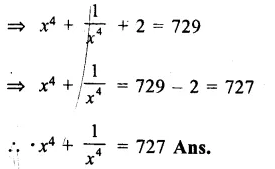Question 13.
Solution:
(i) (x + 1) (x – 1) (x2 + 1)
= {(x)2 – (1)2} (x2 + 1)
= (x2 – 1) (x2 + 1)
= (x2)2 – (1)2 = x4 – 1 Ans.
(ii) (x – 3) (x + 3) (x2 + 9)
= {(x)2 – (3)2 } (x2 + 9)
= (x2 – 9) (x2 + 9)
= (x2)2 – (9)2 = x4 – 81 Ans.
(iii) (3x – 2y) (3x + 2y) (9x2 + 4y2)
= {(3x)2 – (2y)2} (9x2 + 4y2)
= (9x2 – 4y2) (9x2 + 4y2)
= (9x2)2 – (4y2)2
= 81x4 – 16y4 Ans.
(iv) (2p + 3) (2p – 3) (4p2 + 9)
= {(2p)2 – (3)2} (4p2 + 9)
= (4p2 – 9) (4p2 + 9)
= (4p2)2 – (9)2 = 16p4 – 81 Ans.

Question 14.
Solution:
x + y = 12
Squaring both sides,
(x + y)2 = (12)2
=> x2 + y2 + 2xy = 144
=> x2 + y2 + 2 x 14 = 144
=> x2 + y2 + 28 = 144
=> x2 + y2 = 144 – 28 = 116
x2 + y2 = 116 Ans.

Question 15.
Solution:
x – y = 7
Squaring both sides,
(x – y)2 = (7)2
=> x2 + y2 – 2xy = 49
=> x2 + y2 – 2 x 9 = 49
=> x2 + y2 – 18 = 49
=> x2 + y2 = 49 + 18 = 67
x2 + y2 = 67 Ans.

Hope given RS Aggarwal Solutions Class 8 Chapter 6 Operations on Algebraic Expressions Ex 6D are helpful to complete your math homework.

If you have any doubts, please comment below. Learn Insta try to provide online math tutoring for you.